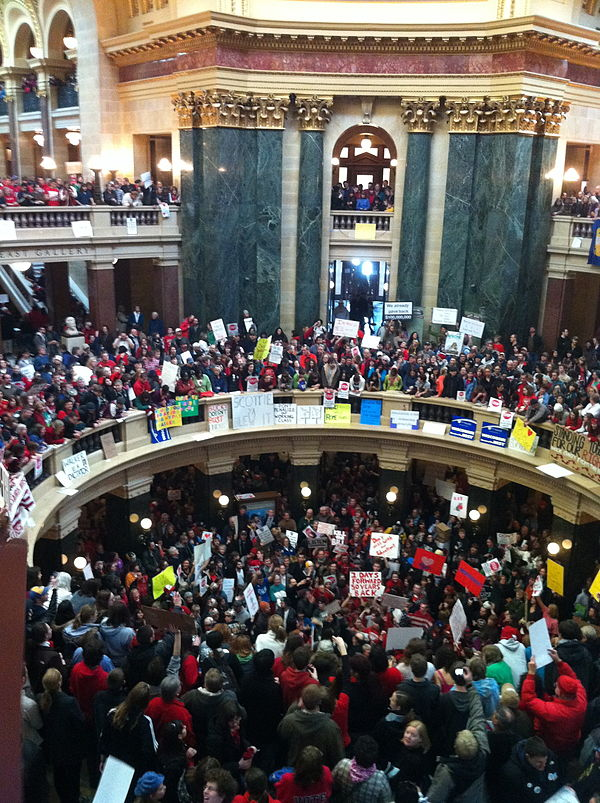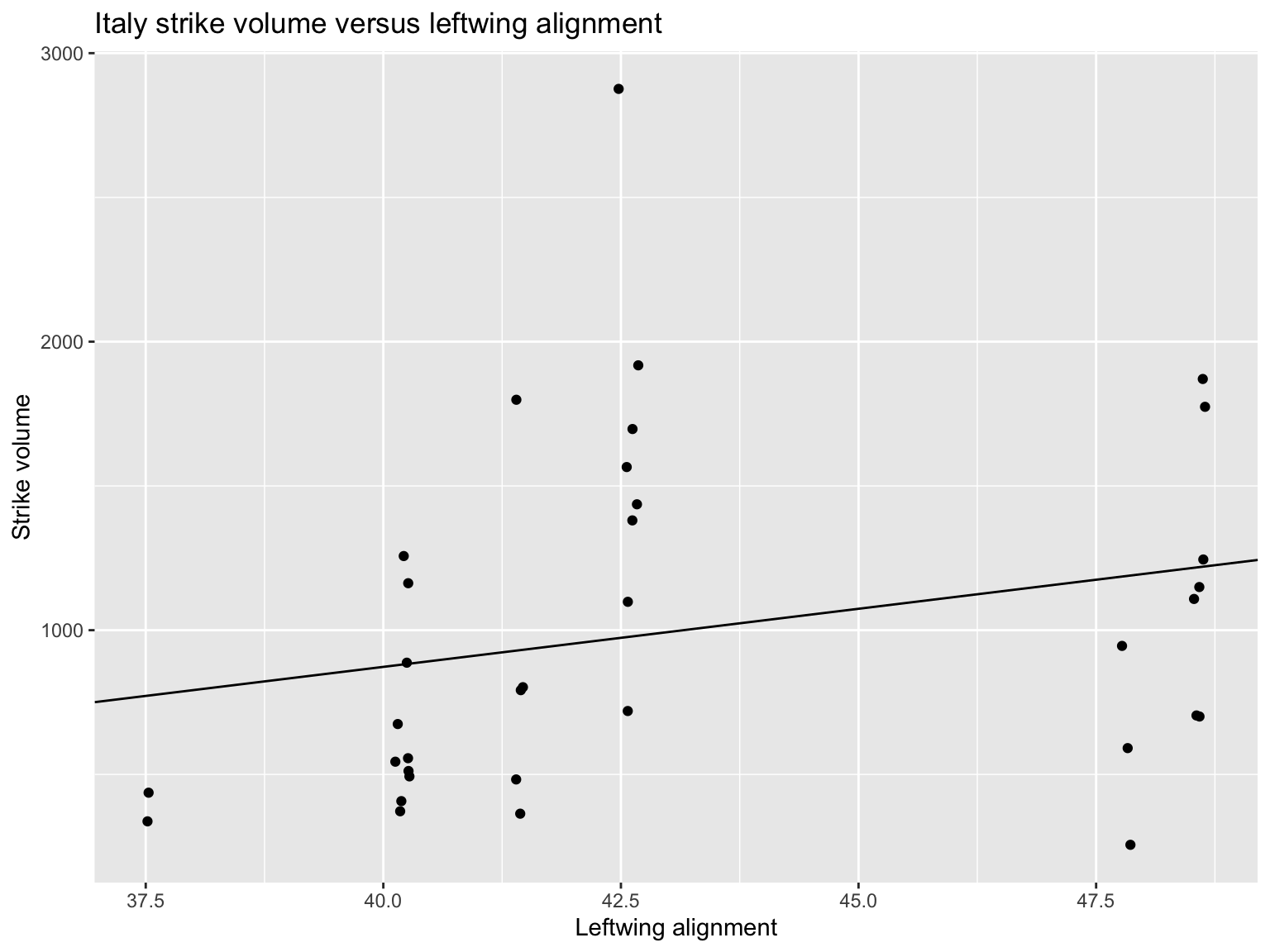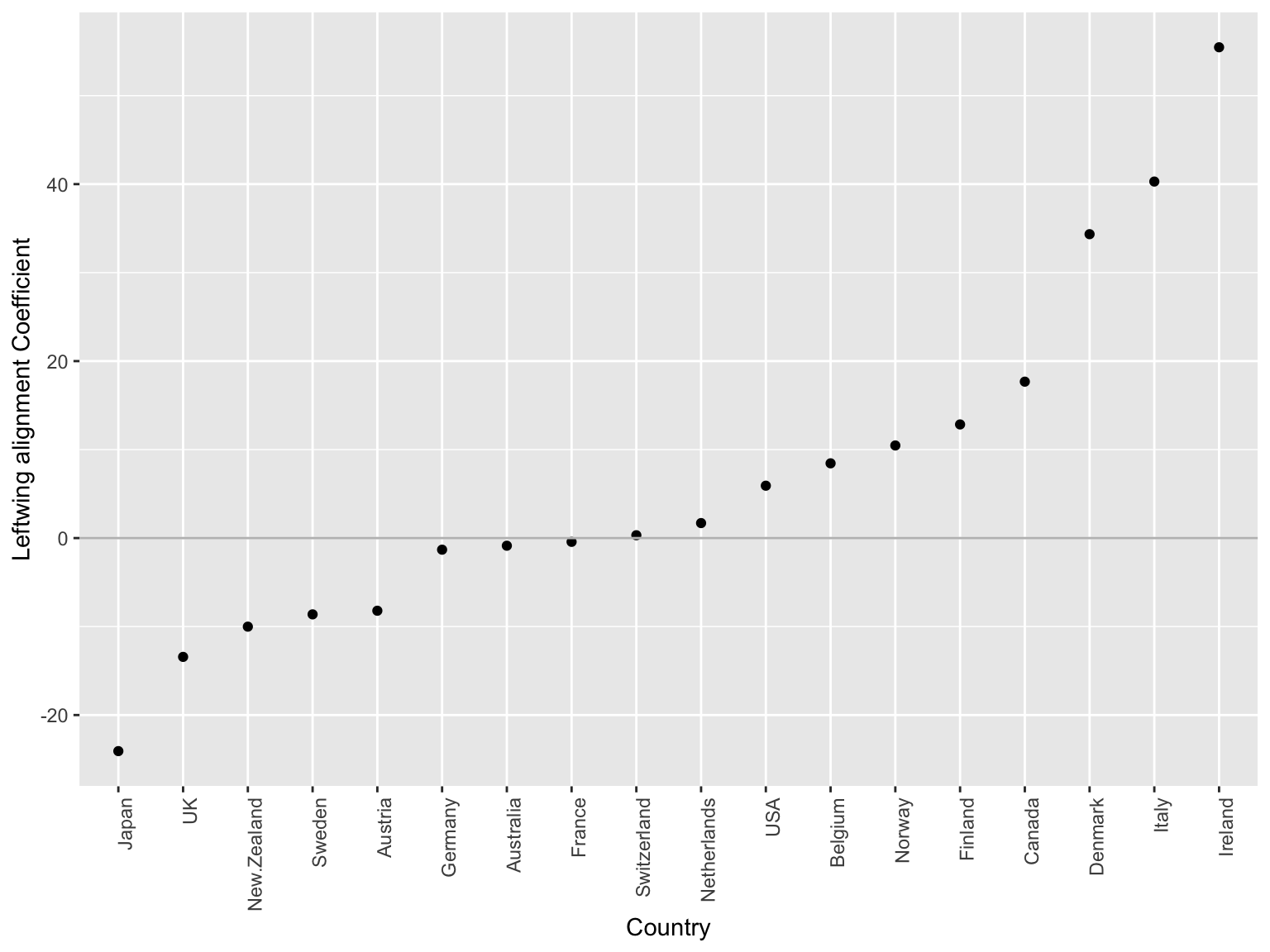Wednesday, August 7, 2019

# Last time: Prediction

• prediction focuses on models with the smallest test / prediction error
• used when you do not know the underlying structure or are just generally interested in prediction - not inference
• Can estimate the test error with cross validation or sample splitting
• training error should not be optimized as it underestimates the test error
• Statistical methods for prediction that are non-parameter include:
• KNN (K - nearest neighbor)
• trees, boosting and random forest
• SVM, kernel machines
• deep learning
• etc…

# Overview for the day:

2. Parallelization
3. Deep Learning

# Review of tidyverse: `dplyr` and `tidyr`

• The tidyverse is a collection of packages for common data science tasks
• Tidyverse functionality is greatly enhanced using pipes (`%>%` operator)
• `dplyr` has advanced functionality that mirrors SQL
• `slice()` and `filter()`: subset rows based on numbers or conditions
• `select()` and `mutate()`: select columns or create/ override columns
• `group_by()`: create groups of rows according to a condition
• `summarize()`: apply computations across groups of rows
• `tidyr` is a package for manipulating the structure of data frames
• `gather()`: make wide data longer
• `spread()`: make long data wider

# Split-apply-combine

Today we will learn a general strategy that can be summmarized in three conceptual steps:

• Split whatever data object we have into meaningful chunks
• Apply the function of interest to each element in this division
• Combine the results into a new object of the desired structure

These are conceptual steps; often the apply and combine steps can be performed in multiple steps (in the `tidyverse` reable fashion), and the `combine` can also see reformating (leveraging `gather` and `spread`)

• As usual, compared to explicit `for()` loops, often requires far less code and more synatically easy to read
• Fits nicely with our previous ideas of top-down function design, in `tidyverse`
• Sets you in the right direction towards learning how to use MapReduce/Hadoop for really, really big data sets
• relative to `tidyverse`: goes much beyond the single number `summarize` that we’ve seen before

# Strikes data set

Data set on 18 countries over 35 years (compiled by Bruce Western, in the Sociology Department at Harvard University). The measured variables:

• `country`, `year`: country and year of data collection
• `strike.volume`: days on strike per 1000 workers
• `unemployment`: unemployment rate
• `inflation`: inflation rate
• `left.parliament`: leftwing share of the goverment
• `centralization`: centralization of unions
• `density`: density of unions
``````library(tidyverse)
dim(strikes_df) # Since 18 × 35 = 630, some years missing from some countries``````
``##  625   8``
``head(strikes_df)``
``````##     country year strike.volume unemployment inflation left.parliament
## 1 Australia 1951           296          1.3      19.8            43.0
## 2 Australia 1952           397          2.2      17.2            43.0
## 3 Australia 1953           360          2.5       4.3            43.0
## 4 Australia 1954             3          1.7       0.7            47.0
## 5 Australia 1955           326          1.4       2.0            38.5
## 6 Australia 1956           352          1.8       6.3            38.5
##   centralization density
## 1      0.3748588      NA
## 2      0.3751829      NA
## 3      0.3745076      NA
## 4      0.3710170      NA
## 5      0.3752675      NA
## 6      0.3716072      NA``````

# An interesting question

Is there a relationship between a country’s ruling party alignment (left versus right) and the volume of strikes?How could we approach this?

• Worst way: by hand, write 18 separate code blocks
• Better Option 1: explicit `for()` loop, where we loop over countries
• Better (Best?) Option 2: use split-combine-apply

# Work with just one chunk of data

Step 0: design a function that will work on one chunk of data

So let’s write code to do regression on the data from (say) just Italy

``````strikes_df_italy <- strikes_df %>% filter(country == "Italy") # Data for Italy
dim(strikes_df_italy)``````
``##  35  8``
``head(strikes_df_italy)``
``````##   country year strike.volume unemployment inflation left.parliament
## 1   Italy 1951           437          8.8      14.3            37.5
## 2   Italy 1952           337          9.5       1.9            37.5
## 3   Italy 1953           545         10.0       1.4            40.2
## 4   Italy 1954           493          8.7       2.4            40.2
## 5   Italy 1955           511          7.5       2.3            40.2
## 6   Italy 1956           372          9.3       3.4            40.2
##   centralization density
## 1      0.2513799      NA
## 2      0.2489860      NA
## 3      0.2482739      NA
## 4      0.2466577      NA
## 5      0.2540366      NA
## 6      0.2457069      NA``````
``````italy_lm <- lm(strike.volume ~ left.parliament, data = strikes_df_italy)
summary(italy_lm)``````
``````##
## Call:
## lm(formula = strike.volume ~ left.parliament, data = strikes_df_italy)
##
## Residuals:
##    Min     1Q Median     3Q    Max
## -930.2 -411.6 -137.3  387.2 1901.4
##
## Coefficients:
##                 Estimate Std. Error t value Pr(>|t|)
## (Intercept)      -738.75    1200.62  -0.615    0.543
## left.parliament    40.29      27.76   1.451    0.156
##
## Residual standard error: 583.3 on 33 degrees of freedom
## Multiple R-squared:  0.05999,    Adjusted R-squared:  0.0315
## F-statistic: 2.106 on 1 and 33 DF,  p-value: 0.1562``````
``````ggplot(strikes_df_italy,
aes(x = left.parliament, y = strike.volume)) +
geom_jitter() +
geom_abline(intercept = coef(italy_lm),
slope = coef(italy_lm)) +
labs(title = "Italy strike volume versus leftwing alignment",
y = "Strike volume", x = "Leftwing alignment")``````# (Continued)

Now let’s turn this into a function

``coef(italy_lm) # Old way``
``````##     (Intercept) left.parliament
##      -738.74531        40.29109``````

Recalling `tidyverse` really likes data.frames we introduce a new package `broom`:

• `broom` converts “tidy”s up different objects (like models) into a `data.frame`s using the `tidy` function
``````my_strike_lm <- function(data) {
coef_table <- lm(strike.volume ~ left.parliament, data = data) %>%
summary() %>%
broom::tidy()
# needs to provide a data frame (to preserve name structure)
return(coef_table %>% select(term, estimate))
}

my_strike_lm(strikes_df_italy) # New way, same results in different format``````
``````## # A tibble: 2 x 2
##   term            estimate
##   <chr>              <dbl>
## 1 (Intercept)       -739.
## 2 left.parliament     40.3``````

# Split our data into appropriate chunks

Step 1: split our data into appropriate chunks, each of which can be handled by our function. Here, the function `nest()` is often helpful: `df %>% group_by(my_factor) %>% nest()` splits a data frame `df` into several data frames, defined by constant levels of the factor `my_factor` and “nests” these data.frames into the “tibble/data.frame” in the data column.

So we want to split `strikes_df` into 18 smaller data frames, each of which has the data for just one country

``````strikes_by_country <-
strikes_df %>% group_by(country) %>%
nest()
class(strikes_by_country) # It's a tibble (just a fancy data.frame)``````
``##  "tbl_df"     "tbl"        "data.frame"``
``names(strikes_by_country) # It has a new column called `data```
``##  "country" "data"``
``head(strikes_by_country, 3)``
``````## # A tibble: 3 x 2
##   country   data
##   <fct>     <list>
## 1 Australia <tibble [35 × 7]>
## 2 Austria   <tibble [35 × 7]>
## 3 Belgium   <tibble [30 × 7]>``````
``head(strikes_by_country\$data[strikes_by_country\$country == "Italy"]) # Same as what we saw before``
``````## []
## # A tibble: 35 x 7
##     year strike.volume unemployment inflation left.parliament
##    <int>         <int>        <dbl>     <dbl>           <dbl>
##  1  1951           437          8.8      14.3            37.5
##  2  1952           337          9.5       1.9            37.5
##  3  1953           545         10         1.4            40.2
##  4  1954           493          8.7       2.4            40.2
##  5  1955           511          7.5       2.3            40.2
##  6  1956           372          9.3       3.4            40.2
##  7  1957           407          7.4       1.1            40.2
##  8  1958           363          6.4       3.2            41.4
##  9  1959           792          5.4       0              41.4
## 10  1960           483          4         2.1            41.4
## # … with 25 more rows, and 2 more variables: centralization <dbl>,
## #   density <dbl>``````

# Apply our function and combine the results

Steps 2 and 3: apply our function to each chunk of data, and combine the results. Here, we’ll apply our functions using `map` from `purrr`.

So we want to apply `my_strikes_lm()` to each subset of the data in `strikes_by_country`. Think about what the output will be from each function call: vector of length 2 (intercept and slope):

``````nested <- strikes_df %>%
group_by(country) %>%
nest() %>%
mutate(coef = map(data, my_strike_lm))

``````## # A tibble: 3 x 3
##   country   data              coef
##   <fct>     <list>            <list>
## 1 Australia <tibble [35 × 7]> <tibble [2 × 2]>
## 2 Austria   <tibble [35 × 7]> <tibble [2 × 2]>
## 3 Belgium   <tibble [30 × 7]> <tibble [2 × 2]>``````

# Unnesting (Combining)

Now, we’re really looking for a data frame that looks like the following:

country (Intercept) left.parliament
Austrialia 415 -0.86
Austria 423 -8.21

So we first just grab the columns of interest (`country` and `coef`) and then “unnest” the nested columns (which in this case is only `coef`).

``````examine_coef <- nested %>% select(country, coef) %>%
unnest()

``````## # A tibble: 4 x 3
##   country   term            estimate
##   <fct>     <chr>              <dbl>
## 1 Australia (Intercept)      415.
## 2 Australia left.parliament   -0.864
## 3 Austria   (Intercept)      423.
## 4 Austria   left.parliament   -8.21``````

This is slightly different than our desired output, but `tidyr::spread` can help us out:

``````strikes_coef <- examine_coef %>% spread(term, estimate)

``````## # A tibble: 2 x 3
##   country   `(Intercept)` left.parliament
##   <fct>             <dbl>           <dbl>
## 1 Australia          415.          -0.864
## 2 Austria            423.          -8.21``````

# Visualize

Now that we’ve create the coefficient matrix, let’s visual the effect of a leftwing leaning parliment on the number of strikes:

``````ggplot(strikes_coef,
aes(x = forcats::fct_reorder(country, left.parliament),
#^ forcats is a great tool to reorder levels of factors
y = left.parliament)) +
geom_point() +
geom_hline(yintercept = 0, color = "grey") +
theme(axis.text.x = element_text(angle = 90, hjust = 1)) +
labs(x = "Country", y = "Leftwing alignment Coefficient")``````# Extra: 1d output Example

• The previous could be seen as “easy” since we had multiple rows, below we do a similar thing to a vector (using `broom::tidy`) to convert it to a `data.frame`.
``````# my inner function
summarize_left_parliament <- function(df) {
broom::tidy(summary(df\$left.parliament))
}

# with the nexted data
summary_lp_nest <- nested %>%
mutate(sum_lp = map(data, summarize_left_parliament))

``````## # A tibble: 2 x 4
##   country   data              coef             sum_lp
##   <fct>     <list>            <list>           <list>
## 1 Australia <tibble [35 × 7]> <tibble [2 × 2]> <tibble [1 × 6]>
## 2 Austria   <tibble [35 × 7]> <tibble [2 × 2]> <tibble [1 × 6]>``````
``````# creating the desired data frame.
summary_lp_unnested <- summary_lp_nest %>% select(country, sum_lp) %>%
unnest
``````## # A tibble: 5 x 7
##   country   minimum    q1 median  mean    q3 maximum
##   <fct>       <dbl> <dbl>  <dbl> <dbl> <dbl>   <dbl>
## 1 Australia    28.3  36.9   41    41.9  47.2    60
## 2 Austria      43.6  46.7   47.9  48.4  50.8    51.9
## 3 Belgium      29.3  30.2   33    35.6  41.6    42.5
## 4 Canada       21.5  51.3   59    55.3  67      78.7
## 5 Denmark      36    44     46.9  46.4  48.8    50.9``````

# Commentary:

1. can also use `split` to put data frames into a list and try doing things like `lapply` (see lectures like: Ryan Tibshirani’s - search “split-apply-combine”.)
2. A lot of things can still intelligently be done using `for`-loops (and thinking about how you need to save your data)
3. in general “split-apply-combine” requires you to think critically about the full process and how you’d like to present the data at the end (which is a very useful skill - knowing multiple ways to do so is every better)

# Part II

Parallel Computing

# Why Parallel Computing

• processing large amounts of data is time consuming.
• a lot of analysis is jsut a set of `for` loops, which do not require the previous to be computed befor the next step

# Why Parallel Computing (Continued)

Much R code runs fast and fine on a single processor. But at times, computations can be:

• cpu-bound: Take too much cpu time
• memory-bound: Take too much memory
• I/O-bound: Take too much time to read/write from disk
• network-bound: Take too much time to transfer

To help with cpu-bound computations, one can take advantage of modern processor architectures that provide multiple cores on a single processor, and thereby enable multiple computations to take place at the same time. In addition, some machines ship with multiple processors, allowing large computations to occur across the entire cluster of those computers. Plus, these machines also have large amounts of memory to avoid memory-bound computing jobs.

# When shouldn’t you parallelize

• parallelization increases overhead and can reduce efficiency
• some task cannot be parallelized

Amdahl’s Law: where the speedup of the computation (y-axis) is a function of both the number of cores (x-axis) and the proportion of the computation that can be parallelized (see colored lines):